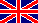VLE-Calc.com Vapor-Liquid Equilibrium CalculatorVersion history

2019

5.0

• New, responsive design
• Azeotrope page moved to azeotrope.info
• Completely rewritten program.
• Introduction of using PSRK equation of state (EOS), when the pressure or the temperature gets somewhat closer to the critical point, or when the mixture contains supercritical compounds.
• Liquid stability (phase-split) is tested by finding all the local minima of the so-called Tangent-Plane-Distance-Function, using the method of Wasylkiewicz et al. This method can detect liquid heterogenity even in the metastable zone, and it can detect, if the system splits into three coexisting liquid phases.
• LLE is calculated by solving the Rachford-Rice equation system (2-phase or 3-phase).
• For ternary VLE calculations the LLE data are precalculated due to the enormous resource requirements of the LLE-engine.
• New, gaseous compounds were added, since they can be handled by the EOS method.
• New VLE diagram type: residue curve set
• Introduction of lots of new bugs so I don't get bored in future developments

2014 - 2015

4.0

• Completely rewritten program.
• Slightly modified user interface for handling of about 200 new compounds.
• Handling of non-ideality changed to UNIQUAC / UNIFAC
• VLE was calculated still by a modified Raoult law.
• Liquid stability (phase-split) was tested by checking the sign of d2Gmix./dx2. (I.e. it did not detect phase split in the metastable region.)
• LLE was calculated by an iso-activity search method using Newton-Raphson root finding. (Still 2-phases only)
• The azeotropic reference-database (experimental/literature data) ceases, from now azeotropic data is predicted by VLE-calculations. From now all the binary and ternary mixture combinations can be checked for azeotropes.
• Extraction calculator page ceases due to lack of interest

2013

3.0

• Introduction of LLE calculations (2-phase only). From now LLE phase diagrams can be plotted too. LLE was calculated using special functions fitted to experimental data.
• From now diagrams are drawn by Google's Visualization API.
• A few more new compounds
• Calculation of simple, single stage extraction process
• Better looking contour lines in the triangle diagrams (finer net and more colors).

2011 - 2013

2.0

• Redesigned user interface, completely rewritten/redesigned program.
• Temperature-dependent non-ideality functions
• Enhanced batch distillation algorithm
• Introduction of continuous ('flash') evaporation
• Introduction of unit conversion
• Isothermal VLE-diagrams
• Plotting triangle VLE-diagrams by contour lines instead of coloured map
• A few more new compounds

2009 - 2011

1.0

• First public version with about a dozen organic solvents
• Showing basic physical data of the substances
• Stored experimental (literature) binary azeotrope data for all combinations, and a few ternary azeotropes
• VLE calculations used a modified Raoult law.
• Non-ideality was handled with special functions fitted to experimental data.
• LLE was not handled at all.
• VLE diagrams were plotted for single compound as well as for binaries and ternaries.
• The diagrams were drawn by Walter Zorn's JavaScript Graphics Library